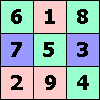ONE TO NINE by Andrew HodgesPage 285: This provocative article by Simon Jenkins makes similar points about education, referring to his own maths courses. I am not a great fan of schools. This article by Johann Hari is a lot more realistic than a great deal of educational theory.

More positively, I was very impressed by the work done in Yorkshire (East Riding) education by a team led by David Mell. Their projects, mainly in primary schools, wove together an appreciation of number together with music and artistic pattern. This fine report produced by David Mell gives many illustrations of the achievements.

Page 293: Fairly daft facts about the number 23.

Page 294:

The first question simply uses the meaning of decimal notation, with its place-notation extended to fractions. The expression .111111... simply means: one-tenth plus one-hundredth plus one-thousandth...

The second question gives another way of seeing why this recurring decimal is equal to 1/9. Use the symbol r for 1/10, then .111111... is written r + r2 + r3 + r4...

Multiply this by (1 − r) and get ( r + r2 + r3 + r4...) − (r2 + r3 + r4 ...) = r

Hence the recurring decimal is r/(1 − r) = (1/10)/(1 − 1/10) = 1/9

Page 298:

This problem shows another way of seeing why the recurring decimal for 1/7 is right. This time let r = 10−6, and then the same argument shows that

1/999999 = 10−6 + 10−12 + 10−18... = .000001000001000001000001000001...

Multiplying by 142857:

142857/999999 = 1/7 = .142857142857142857142857...

Page 301

1/11 = .09090909...
1/37 = .027027027...
1/101 = .009900990099...
1/41 = .0243902439...

These fit the rule because 2 divides into 10, 3 divides into 36, 4 divides into 100, and 5 divides into 40.

Page 302:

It is easy to see the every remainder has a unique successor. If the remainder is r, then its successor is just the remainder when r × 10 is divided by p.

To see that each remainder has a unique predecessor, suppose if possible that there are two remainders s and t which both have r as their successor. Then r is the remainder when s × 10 is divided by p, and also the remainder when t × 10 is divided by p. Then the number (s − t) × 10 must be divisible by p. But this is impossible, as p is a prime greater than 5.

This establishes that the remainders must form cycles, or carousels. But there will in general be several disjoint carousels. We want to show they all have the same length. This is most easily seen from the example of 1/13, which has the two cycles 1-10-9-12-3-4 and 2-7-5-11-6-8. The second cycle can be obtained from the first by multiplying each element by 2, modulo 13. Thus it must have the same length. The same argument applies in general.Page 306:

75025 and 46368 give an example of where the Euclidean algorithm makes the slowest progress, because they are Fibonacci numbers. The quotients are always 1 so the reduction is very slow.

75025 = 1 × 46368 + 28657 reducing to (46368, 28657)
46368 = 1 × 28657 + 17711 reducing to (28657, 17711)
28657 = 1 × 17711 + 10946 reducing to (17711, 10946)
...and so on through all the Fibonacci numbers to
13 = 1 × 8 + 5 reducing to (8, 5)
8 = 1 × 5 + 3 reducing to (5, 3)
5 = 1 × 3 + 2 reducing to (3, 2)
3 = 1 × 2 + 1 reducing to (2, 1).Page 310:

The question of non-unique representation in base-2 fractions is just the same as we met with 1 = .99999999 recurring in base-10 decimals. For the same reason, in binary,

a half is .10000000... but also .01111111...

The same applies to any number which has the form of an integer divided by some power of two. There are infinitely many such numbers, but it is the same infinity as the number of integers.

Page 313: Cool video of Mandelbrot set, with song about Mandelbrot.

Ray Girvan gives an astounding account of how the Mandelbrot set was anticipated in the thirteenth century!